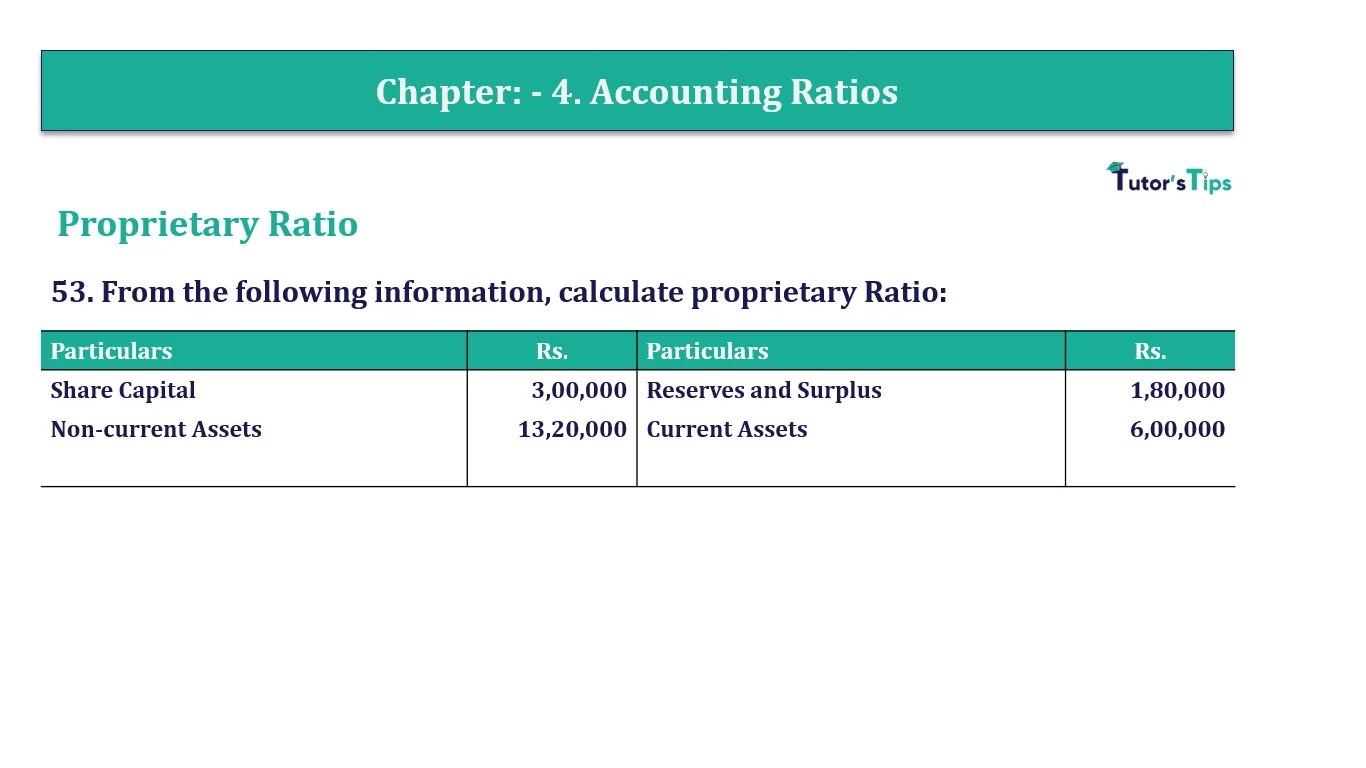# Question 53 Chapter 4 of +2-B – T.S. Grewal 12 ClassQuestion No. 53- Chapter No.4 - T.S. Grewal +2 Book Part B

Question 53 Chapter 4 of +2-B

Proprietary Ratio

53. From the following information, calculate proprietary Ratio:

 Particulars Rs. Particulars Rs. Share Capital 3,00,000 Reserves and Surplus 1,80,000 Non-current Assets 13,20,000 Current Assets 6,00,000

### The solution of Question 53 Chapter 4 of +2-B: –

 Shareholders’ Funds = Share Capital + Reserves and Surplus = Rs. 3,00,000 + Rs. 1,80,000 Shareholders’ Funds = Rs. 4,80,000 Total Assets = Non-current Assets + Current Assets = Rs. 13,20,000 + Rs. 6,00,000 Total Assets = Rs. 19,20,000

 Total Assets to Debt Ratio = Total Assets = Rs.4,80,000 Long- term Debts Rs.19,20,000 = 0.25 : 1

Balance Sheet: Meaning, Format & Examples

Comment if you have any question.

Also, Check out the solved question of previous Chapters: –